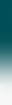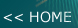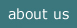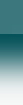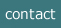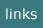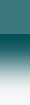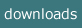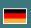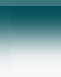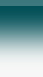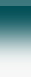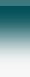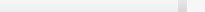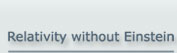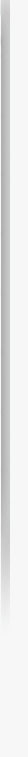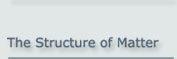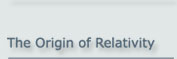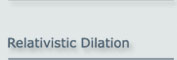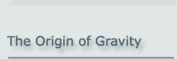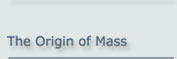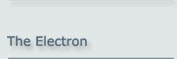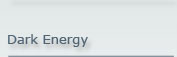Relativity without Einstein
- Relativity without Space-Time -

## It’s true! The concept of 4-dimensional space-time is by no means necessary in order to explain the physics of relativity!

Yes, relativity exists in our physical world. – But do we need Einstein?

What we call RELATIVITY comprises the following measurable phenomena:

For Special Relativity:

(1)     The speed of light ‘c’ is a constant (at least as the result of a measurement)
(2)     Physical objects contract when in motion
(3)     The speed of clocks slows down in motion (and so do all temporal processes)
(4)     Mass increases - which implies mass-energy equivalence

For General Relativity:

(5)    The speed of light ‘c’ is reduced in a gravitational field
(6)     A light beam is curved in a gravitational field
(7)     The speed of clocks is reduced in a gravitational field (and so are all temporal processes)

Einstein explained the phenomena of relativity by assuming that space and time form a close unity, which is described by the 4-dimensional geometry of “space-time”. In the general case, this 4-dimensional system is curved.

The mathematical treatment of a 4-dimensional curved space is a highly challenging task. Only few physicists are able to do this. (Nobel prize-winner Steven Weinberg suspects that there may be fewer than 10 in the whole world.)

However, the same results as those from Einstein’s space-time can be achieved using standard mathematics (even at a school level). – The purpose of this website is to demonstrate this.

As a result, relativity - special as well as general - is not restricted to a few exceptional individuals but can be taught even at school.

How does this work?

Einstein’s assumptions regarding space-time can be replaced by the following facts, which exist independently of relativity:

1. The contraction is simply a consequence of the fact that the fields which hold together the constituents of physical objects contract. The reason for this is the finite speed of light at which the binding fields propagate when in motion.

This consequence was noted very soon after the Michelson-Morley experiment by several physicists (e.g. Heaviside, Fitzgerald, Lorentz), who drew on Maxwell’s recently developed theory of electromagnetism. Meanwhile it has been shown that the contraction occurs for any kind of field.

2. The dilation of any periodic process (such as a clock function) in motion is an immediate consequence of the internal oscillation of elementary particles. The constituents of elementary particles are mass-less and oscillate internally at the speed of light.

This behaviour of elementary particles was basically found by Louis de Broglie in 1923 when he detected the wave properties of elementary particles. A quantitative description was given by Paul Dirac in 1928 when he developed the famous Dirac equation for the electron. Erwin Schrödinger called this motion “Zitterbewegung”.

3. The mass of elementary particles is itself also a consequence of the finite speed of light, at which the fields binding the constituents of the elementary particles propagate.

This mechanism also causes the increase in mass when an object is in motion, which is a direct consequence of the contraction. And this in turn leads directly to Einstein’s famous mass-energy equivalence equation.

For details of this process please refer to the origin of relativity and the origin of mass.

1. The reduction of the speed of light in a gravitational field is a proven fact. It can be explained by a physical model which refers to the assumption of exchange particles that mediate every force.

When a light-like particle interacts with the exchange particles of any force, its motion is inhibited. This causes a reduction in its speed which can be quantitatively explained by this process.

2. The bending of a light-like particle in a gravitational field is a simple classical refraction process.

The stronger the gravitational field is, i.e. the closer a light-like particle is to a gravitational source, the more the speed of light is reduced. This fact causes classical refraction. The numerical result of this refraction conforms exactly to the curvature assumed by Einstein.

3. Gravitational acceleration – corresponding to gravitational mass – is the refraction of the internal motion in an elementary particle.

The oscillating constituents of an elementary particle are also refracted by the gravitational field. The elementary particle itself is thereby caused to move towards the gravitational source. This fact quantitatively explains Newton’s law of gravity in the classical case and General Relativity in the relativistic case.

For details of this process, please refer to the origin of gravity.

The Michelson-Morley experiment and Einstein’s reaction to it

First of all, there is a frequent but fundamental misunderstanding about the Michelson-Morley experiment that is incorrectly propagated even in university lectures: this experiment did not disprove the existence of an ether. It only showed that an ether cannot be measured – a view that was also accepted by Einstein .

Oliver Heaviside and Hendrik Lorentz were able to show that the null result of Michelson and Morley is caused by the contraction of fields in motion and, as a consequence, the contraction of objects like the Michelson-Morley apparatus.

Einstein accepted this explanation logically but did not follow it in constructing his theory. Einstein’s reason was that he adhered to the philosophy of positivism in his early years, which demanded that a theory should not involve invisible phenomena such as the ether . So Einstein insisted on a theory that did not use an ether.

The path chosen by Einstein looks very elegant at first sight. However, it caused logical conflicts which could only be resolved by using this 4-dimensional construct of space-time. – This was a very high price to pay, as the theory developed from these assumptions is very (and unnecessarily) difficult to handle.

This site as pdf file.

Diese Seite auf deutsch.

 A. Einstein, Zur Elektrodynamik bewegter Körper, Annalen der Physik, IV. Jg. 17, S. 891–921 (1905)
 K. R. Popper, Logik der Forschung, J-C.B-Mohr, Tübingen, (1982); (English: The Logic of Scientific Discovery)

2016-05-30